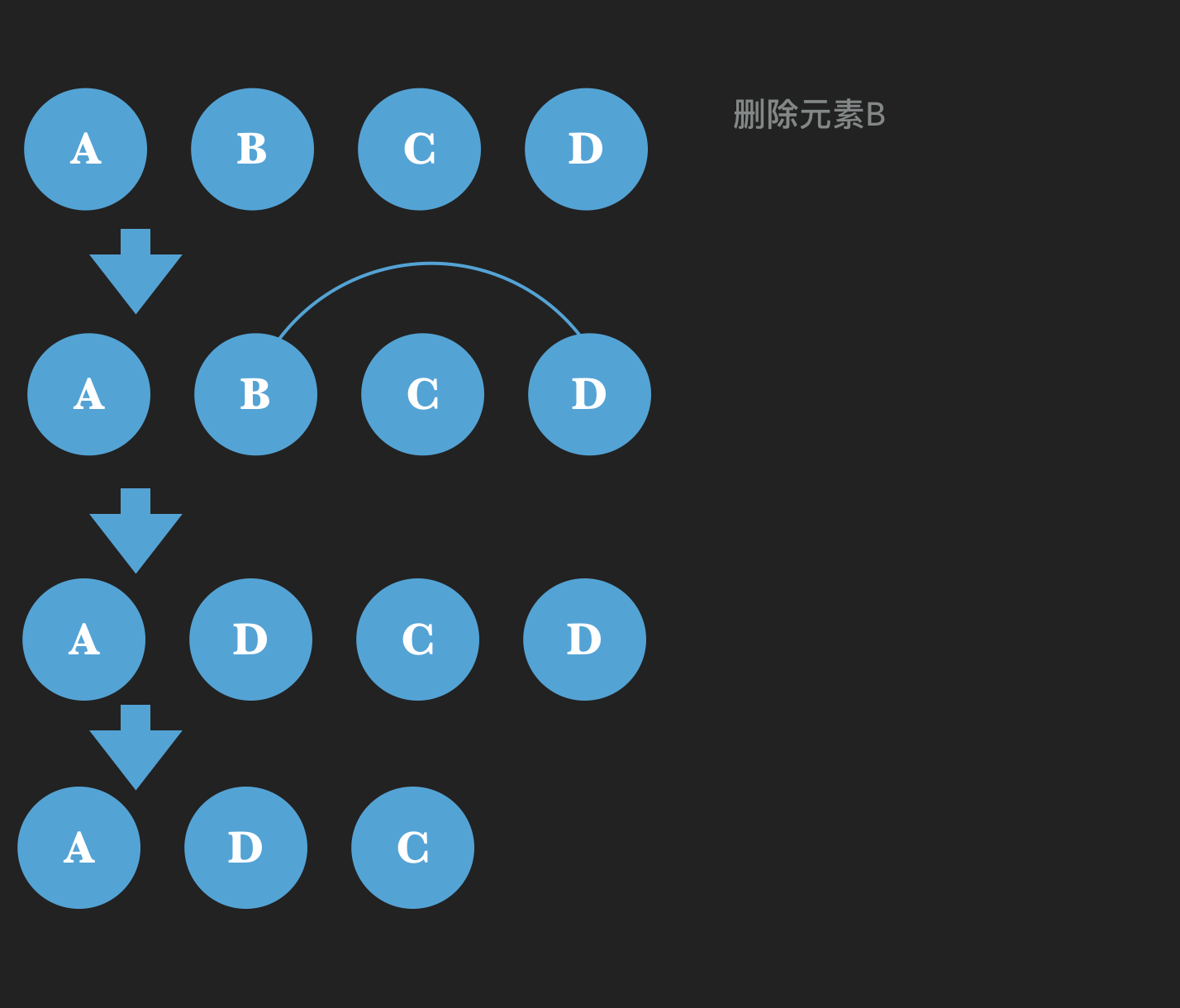# 散列表的原理和应用

### 问题描述

1. 插入操作：询问盒子中是否存在数 x，如果不存在则把数 x 丢到盒子里。
2. 删除操作：询问盒子中是否存在数 x，如果存在则取出 x。

### 样例输入

6
1 100
1 100
2 100
1 200
2 100
2 200


Succeeded
Failed
Succeeded
Succeeded
Failed
Succeeded

#include<iostream>
#include<vector>
using namespace std;
typedef long long ll;
const int Mod = 1000003;
vector<ll> table[Mod];
int has(int x){
return x%Mod;
}
bool check(int op, ll x)
{
int h = has(x);
vector<ll>::iterator ptr = table[h].end();
for(vector<ll>::iterator it = table[h].begin(); it!=table[h].end(); it++ )
{
if( *it == x)
{
ptr = it;
break;
}
}
if( op == 1){ //插入操作
if( ptr!= table[h].end()) //如果已经有这个元素了
{
return 0;
}
else//没有的话
{
table[h].push_back(x);
return 1;
}
}else{ //删除操作
if( ptr != table[h].end())//如果有这个元素
{
*ptr = table[h].back(); //将这个元素被向量最后一个元素替代
table[h].pop_back();
return 1;
}
else
return 0;
}
}
int main()
{
int n; scanf("%d",&n);
int op; ll x;
while(n--)
{
scanf("%d %lld",&op,&x);
if( check(op, x)){
printf("Succeded\n");
}
else{
printf("Failed\n");
}
}
return 0;
}01-231万+
06-0319万+06-04106
12-23818
12-01126
10-205310
01-201800
07-102万+
02-19526